# Workflow in `briskaR`

This vignette explains how to use the `briskaR` package.

The R package `briskaR` offers a generic framework for the simulation of spatially-explicit exposure-hazard model for ecotoxicological studies.

The modeling framework has four main components:

1. Landscape: a set of sources (e.g., polygons of GM fields) and a set of receptors (e.g., lines, points or polygons of host plant areas),
2. Contaminant in the environment: a set of raster maps of concentration of pollen in space and time. The dispersal of contaminants is modeled as a convolution between the emitting functions of GM-pollen from sources and the dispersal kernel. This component also includes the deposition, adherence and loss processes of pollen on leaves, which drive the temporal dynamics of exposure to Bt toxin.
3. Exposed individuals: the location of Non-Target Lepidoptera individuals in the landscape and their phenology. It may include a temperature growth development model.
4. Ecotoxicology: the toxicokinetic-toxicodynamic models (TK-TD) describe (i) the toxicokinetic part describing the temporal dynamics of the internal concentration of the contaminant, that is a proxy of internal concentration to be further used for address lethal and sub-lethal effects, and (ii) the toxicodynamic part describing the impact of the toxicokinetic latent-variables on the fitness of individuals, lethal or sublethal.

A simulation run is divided in 7 steps:

1. Landscape
• Source: landscape patches of GM field locations
• Host: landscape patches of potential Non-Target Lepidoptera laying sites
1. Dispersal
• Kernel: probability distribution of pollen dispersal for each GM patches
1. Convolution
• Emission profile: time profile of emission of pollen for each maize unit
• Convolution of dispersal kernel with emission profile
• Deposition: adherence of pollen on leaves ; loss of pollen due to rain washing of leaves
1. Habitat
• Location: Laying site of Non-Target Lepidoptera
• Emergence: Date of emergence of larvae
1. Phenology
• Development: dynamics of larvae along time: emergence, survival and reproduction
1. Exposure
• Overlapping: exposure of larvae time-line to pollen deposition
1. Damage
• Survival: effect of Bt-toxin exposure on larval survival
• Reproduction: effect of Bt-toxin exposure on reproduction

# Landscape

``````data("sfMaize65")

# PLOT ------------------------------------------------------------------------
ggplot() + theme_minimal() +
scale_fill_manual(values = c("grey", "orange"),
name = "Maize") +
geom_sf(data = sfMaize65,
aes(fill = as.factor(maize)))``````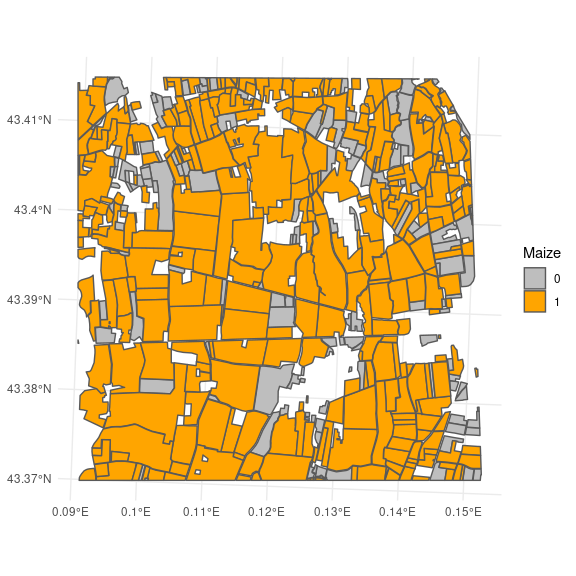``````# Random 20% are GM field
sfMaize65\$maize_GM <- sfMaize65\$maize * rbinom(n = nrow(sfMaize65), size = 1 , prob = 0.2)``````
``````# filter table to have only GM fields
sfMaize65_GM <- sfMaize65[sfMaize65\$maize_GM == 1,]

## PLOT ------------------------------------------------------------------------
plt_GM <- ggplot() + theme_minimal() +
scale_fill_manual(values = c("grey", "red"),
name = "GM maize") +
geom_sf(data = sfMaize65,
aes(fill = as.factor(maize_GM)))
plt_GM +
geom_sf_text(data = sfMaize65_GM,
aes(label = label))``````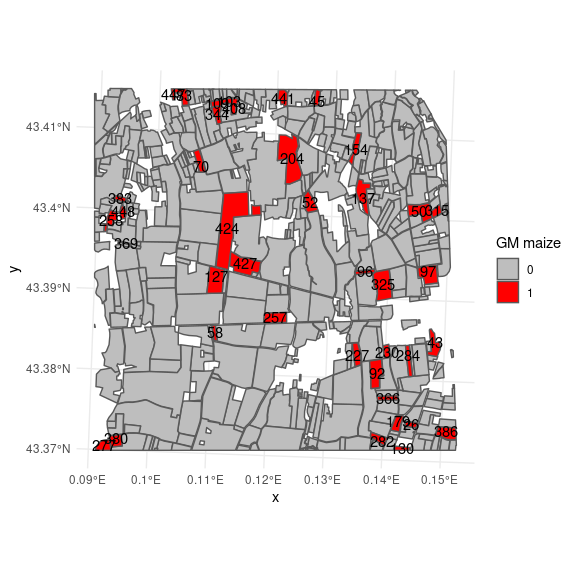Before proceding the dispersal, we can add a buffer around the landscape. A good approach would be to define sources and receptors at this step in order to embedded all area within the square frame. The eposure is going to be computed over this square frame.

``````squareFrame_sfMaize65 <- st_squared_geometry(list(sfMaize65), buffer = 200)

# PLOT ------------------------------------------------------------------------
plt_GM +
geom_sf(data = squareFrame_sfMaize65, fill = NA)``````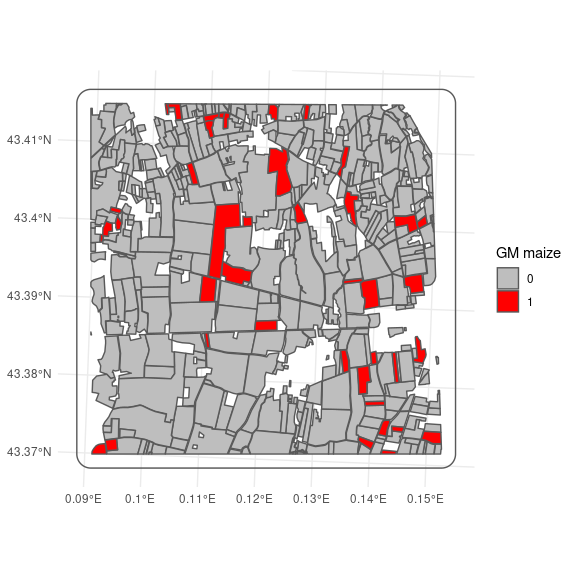``````stack_dispersal <- brk_dispersal(sfMaize65_GM,
size_raster = 2^8,
kernel = "geometric",
kernel.options = list("a" =  -2.63),
squared_frame = squareFrame_sfMaize65)``````
``## Starting dispersal computing...``
``## Raster size : 2.56e+02``
``````## Step 1/2: Rasterize... fini
## Step 2/2: Compute field... 26... 43... 45... 50... 52... 58... 70... 83... 92... 96... 97... 103... 109... 127... 130... 137... 154... 179... 204... 227... 230... 257... 258... 277... 282... 284... 315... 325... 344... 366... 369... 380... 383... 386... 408... 424... 427... 441... 447... 448... fini``````
``````# PLOT ------------------------------------------------------------------------
raster::plot(stack_dispersal[[1:6]])``````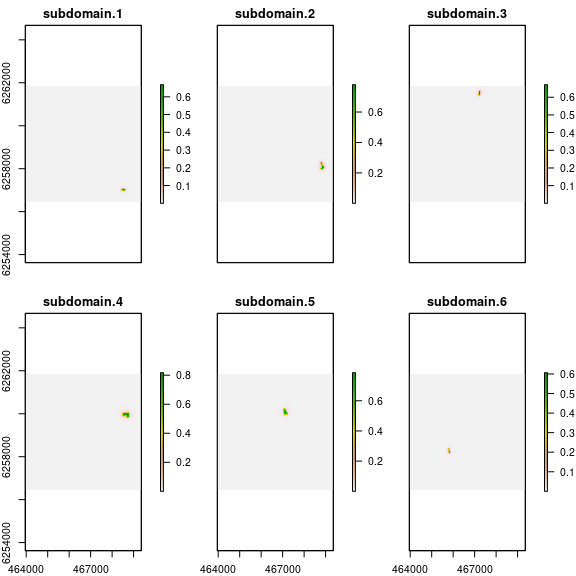## Emission

Emission, Exposure, and then individuals developement, migration and so need a time line. This is done using the function `brk_timeline` as follow:

``````sfMaize65_GM_Pollen <- brk_timeline(sf = sfMaize65_GM,
key = "timeline",
from = as.Date("01-07-2018", format = "%d-%m-%y"),
to = as.Date("01-09-2018", format = "%d-%m-%y"),
by = "days")``````
``````# Profile of emission:
data("maize.proportion_pollen")
graphics::plot(maize.proportion_pollen, type= "l")``````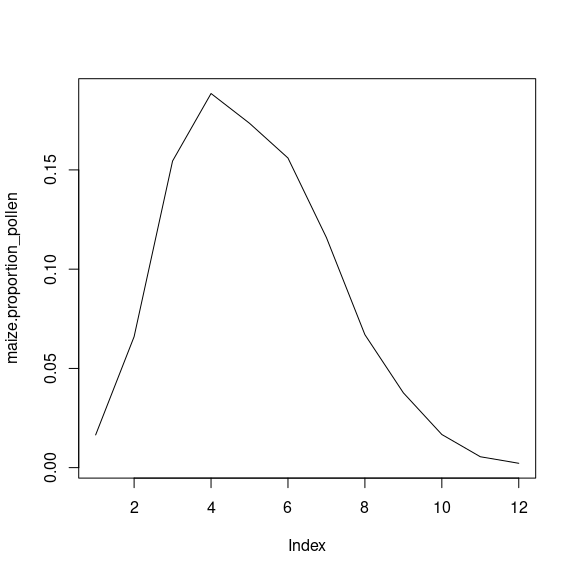``````funTimePollen <- function(time){
density = runif(1, 7, 11)
pollen = rgamma(1, shape = 1.6, scale = 1 / (2 * 10 ^ -7))
nbr_days = length(time)
deb = sample(1:(nbr_days - length(maize.proportion_pollen)), 1)
end = (deb + length(maize.proportion_pollen) - 1)
pollen_emission <- rep(0, nbr_days)
pollen_emission[deb:end] <- as.numeric(pollen * density * maize.proportion_pollen)
return(pollen_emission)
}

sfMaize65_GM_Pollen <- brk_emission(sf = sfMaize65_GM_Pollen,
keyTime = "timeline", # Length of the reference timeline
key = "EMISSION", # Name of the new column
# function over each line of sf
FUN = function(i){
funTimePollen(sfMaize65_GM_Pollen\$timeline[[i]])
})``````
``````## Warning in brk_emission(sf = sfMaize65_GM_Pollen, keyTime = "timeline", : The
## column variable timeline may have changed``````

## Deposition

``````stackTimeline = seq(from = min(do.call("c", sfMaize65_GM_Pollen[["timeline"]])),
to = max(do.call("c", sfMaize65_GM_Pollen[["timeline"]])),
by = "days")

stack_exposure <- brk_exposure(stack_dispersal,
sfMaize65_GM_Pollen,
key = "EMISSION", # Name of the new column
keyTime = "timeline", # Length of the reference timeline
loss = 0.1,
beta = 0.2,
quiet = TRUE)``````
``## Step 1/2: Compute global spatio-temporal exposure profile...``
``## fini``
``## Step 2/2: Convert all matrices to rasters... fini``
``````# PLOT ------------------------------------------------------------------------
raster::plot(stack_exposure[[1:6]])``````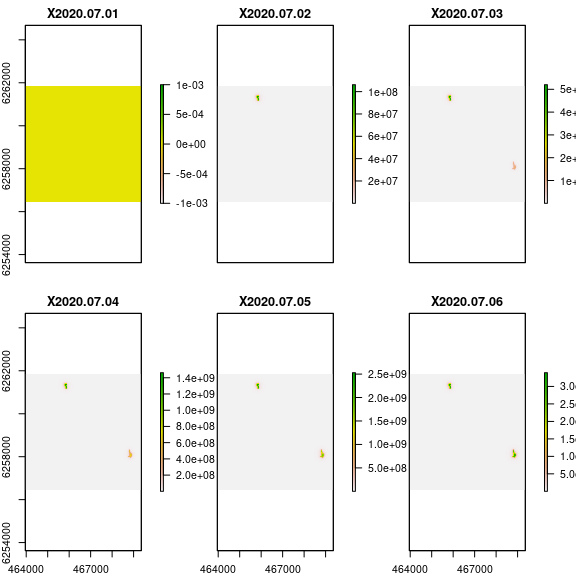Plot of exposure out of GM fields

``````# A MULTIPOLYGON OUT OF GM FIELDS
sfMaize65_outGM <- st_sf(geometry = st_difference(x = st_geometry(squareFrame_sfMaize65),
y = st_union(st_geometry(sfMaize65_GM))))

# POINTS OUT OF GM FIELDS
gridPOINT_squareFrame <- st_make_grid(squareFrame_sfMaize65, n = 30, what = "centers") # grid n x n !!
gridPOINT_outGM <- st_sf(geometry = st_intersection(x = st_geometry(gridPOINT_squareFrame),
y = st_union(st_geometry(sfMaize65_outGM))))

# PLOT ------------------------------------------------------------------------
ggplot() + theme_minimal() +
geom_sf(data = sfMaize65_outGM, fill = "grey30") +
geom_sf(data = gridPOINT_outGM) # point out of GM field``````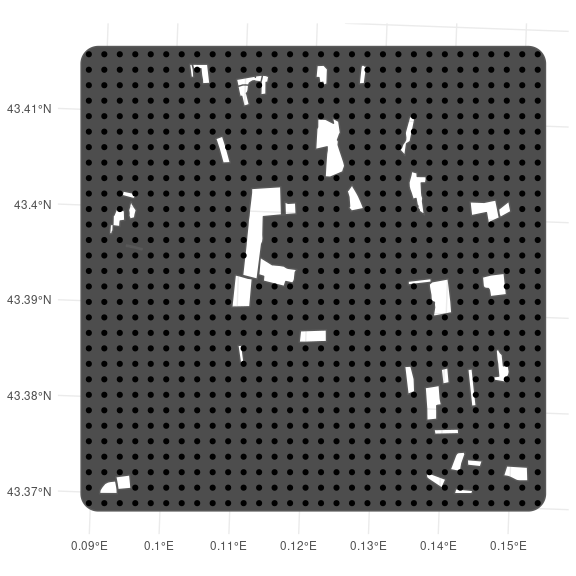``````# Create raster
df_outGM = as.data.frame(raster::extract(x = stack_exposure,
y =  sf::as_Spatial(gridPOINT_outGM)))``````
``````## Warning in showSRID(uprojargs, format = "PROJ", multiline = "NO"): Discarded datum Unknown based on GRS80 ellipsoid in CRS definition,
##  but +towgs84= values preserved``````
``````## Warning in showSRID(SRS_string, format = "PROJ", multiline = "NO"): Discarded datum Unknown based on GRS80 ellipsoid in CRS definition,
##  but +towgs84= values preserved``````
``````#colnames(df_outGM) = paste0("Time_", gsub("-", "\\1", stackTimeline))

sf_outGM = st_as_sf(geometry = st_geometry(gridPOINT_outGM), df_outGM)

# PLOT ------------------------------------------------------------------------
ggplot() + theme_minimal() +
labs(title = paste("Exposure at", colnames(df_outGM))) +
scale_color_continuous(low = "green", high = "red",
trans = "log", name = "log scaled") +
geom_sf(data = sf_outGM,
aes(color = df_outGM[,40]))``````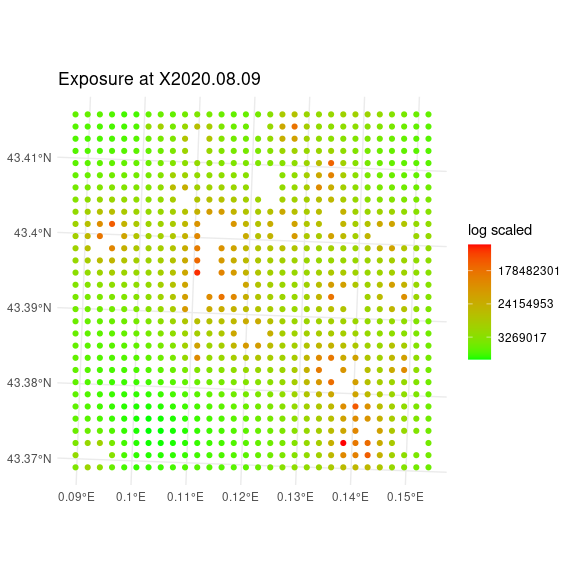# Individuals

## Receptor area

First of all is to define the receptor area.

We can take any set of place within the area where exposure has been computed. A good way is to consider both receptor and source at the beginning in order to embed both in `squareFrame`

``````# Margins around each fields
sfMaize65_receptor = st_multibuffer(sfMaize65_GM,
dist = rep(100, nrow(sfMaize65_GM)))

# PLOT ------------------------------------------------------------------------
plt_GMreceptor <-
ggplot() + theme_minimal() +
geom_sf(data = sfMaize65_GM,
fill = "red") +
geom_sf(data = sfMaize65_receptor,
fill = "#669900")
plt_GMreceptor``````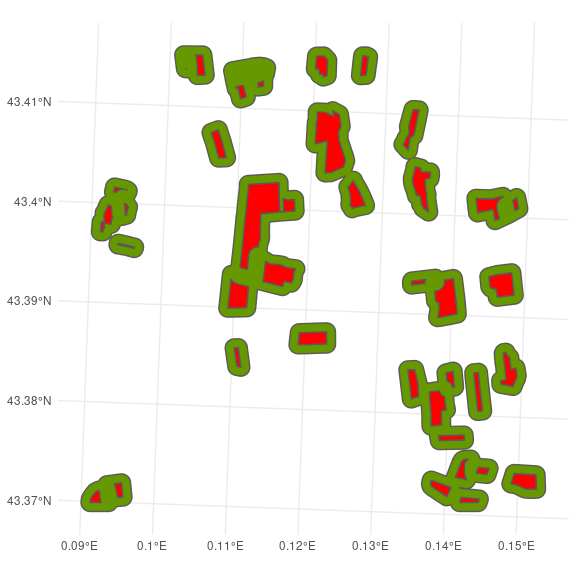## Phenology

### Time-space location of first individuals

``````# 1. Number of site for the first generation
nbrSite = 100
# 2. Set eggs
sfLarvae <- brk_newPoints(sf = sfMaize65_receptor, size = nbrSite) # size = number of sites

# PLOT ------------------------------------------------------------------------
plt_GMreceptor +
geom_sf(data = sfLarvae)``````